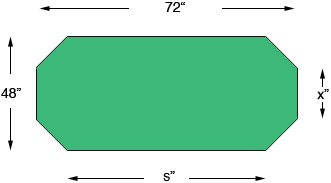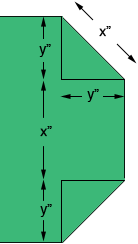Name: Robert Who is asking: Parent Level of the question: Secondary Question: I am building a poker table which is in the shape of an irregular octagon. I know the table measures 72 inches long and 48 inches wide with two parallel straight sides of equal length and six smaller sides of equal length ( three at each end of the table), what I don't know are the lengths of the any of the sides. Robert, Her is what I understand as your table top.I let the long side length by s inches and each of the six short side lengths be x inches. Here is a closer look at one of the ends.The triangle shown is a right triangle and hence, by Pythagoras Theorem, y2 + y2 = x2 or 2 y2 = x2 and hence y = x/√2 Thus the width of the table, 48 inches, can be written 48 = y + x + y = x/√2 + x + x/√2 = (1 + 2/√2 ) x = (1 + √2) x Hence x = 48/(1 + √2) = 19.88" or 19 7/8 inches Thus the length of the long side is s = 72 - 2(19 7/8 ) = 32 1/4 inches. Harley